# SSAT Elementary Level Math : Plane Geometry

## Example Questions

← Previous 1 3 4 5 6 7 8 9 67 68

### Example Question #1 : How To Find The Area Of A Square

What is the area of a square with sides 13 inches long?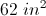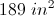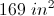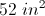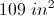Explanation:

The area of a square can be found by multiplying the length of a side by itself: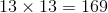### Example Question #1 : Plane Geometry

What is the area of a square that has a side measuring 1 inch in length?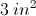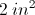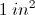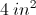Explanation:

A square has four equal sides. The area of a square is found by the equation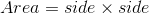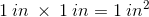### Example Question #1 : How To Find The Area Of A Square

What is the area of a square that has a side measuring 3 inches in length?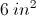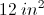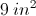Explanation:

A square has four equal sides. The area of a square is found by the equation.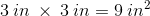### Example Question #1 : Plane Geometry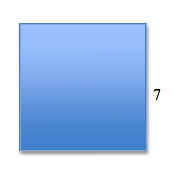What is the area of the square?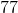Explanation:

To find the area of a square, multiply one side by itself.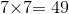### Example Question #1 : How To Find The Area Of A Square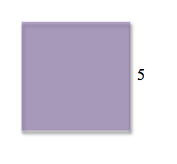What is the area of the square?Explanation:

The area of a square is equal to the length of one side multiplied by itself.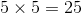### Example Question #1 : How To Find The Area Of A Square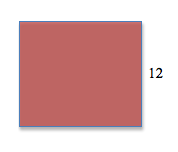What is the area of the square?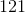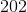Explanation:

To find the area of a square, simply multiply the length of one side by itself!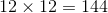### Example Question #1 : How To Find The Area Of A Square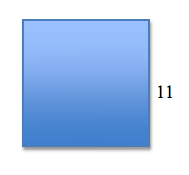What is the area of the square?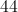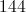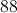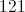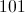Explanation:

To find the area of this square, simply square the side length:### Example Question #1 : How To Find The Area Of A Square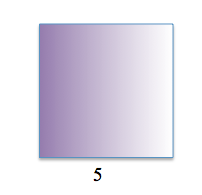What is the area of the above square?Explanation:

To find the area of the square, multiply the length of one side () by itself.### Example Question #1 : Quadrilaterals

What is the AREA of the square in the figure?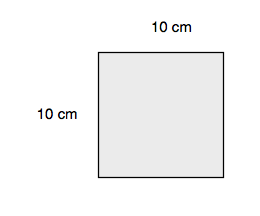40 cm

10 cm

1,000 cm

100 cm

20 cm

100 cm

Explanation:

Because the question asks you to find the AREA of the square, you are looking to figure out how much space the square covers. You find the area of squares by multiplying the length of the square times the width. So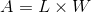, or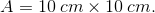Therefore the correct answer is 100 cm.

### Example Question #1 : Squares

A square has a perimeter ofunits. What is the area of the square?units squared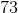units squaredunits squaredunits squaredunits squaredunits squared
Since a square has four sides of the same length, you can divide its perimeter byto figure out the length of one of its sides:units. The area of a square can be calculated by multiplying two of its sides together, so the area of this square isunits squared, because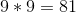.